出租广告位,需要合作请联系站长

+关注

2020-07(10)

2020-08(50)

## 1.用数据做判断：布尔值

True和False，叫做【布尔值】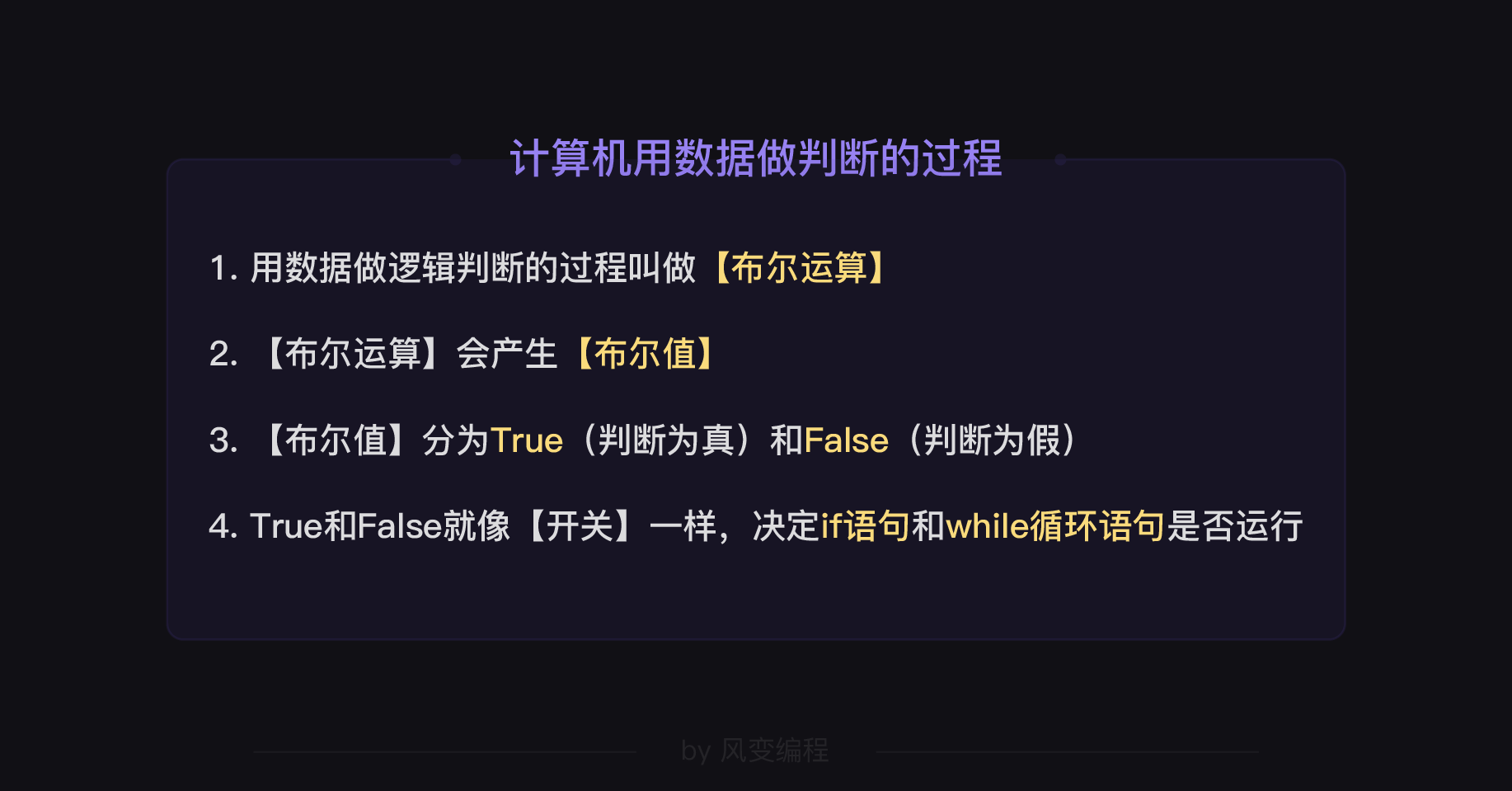## 2.两个数值做比较

``````password = input('请输入密码：')
print('密码正确！')
else:
print('密码错误！')
``````

## 3.直接用数值做运算

``````if 1:
print('熊猫')
>>>熊猫
``````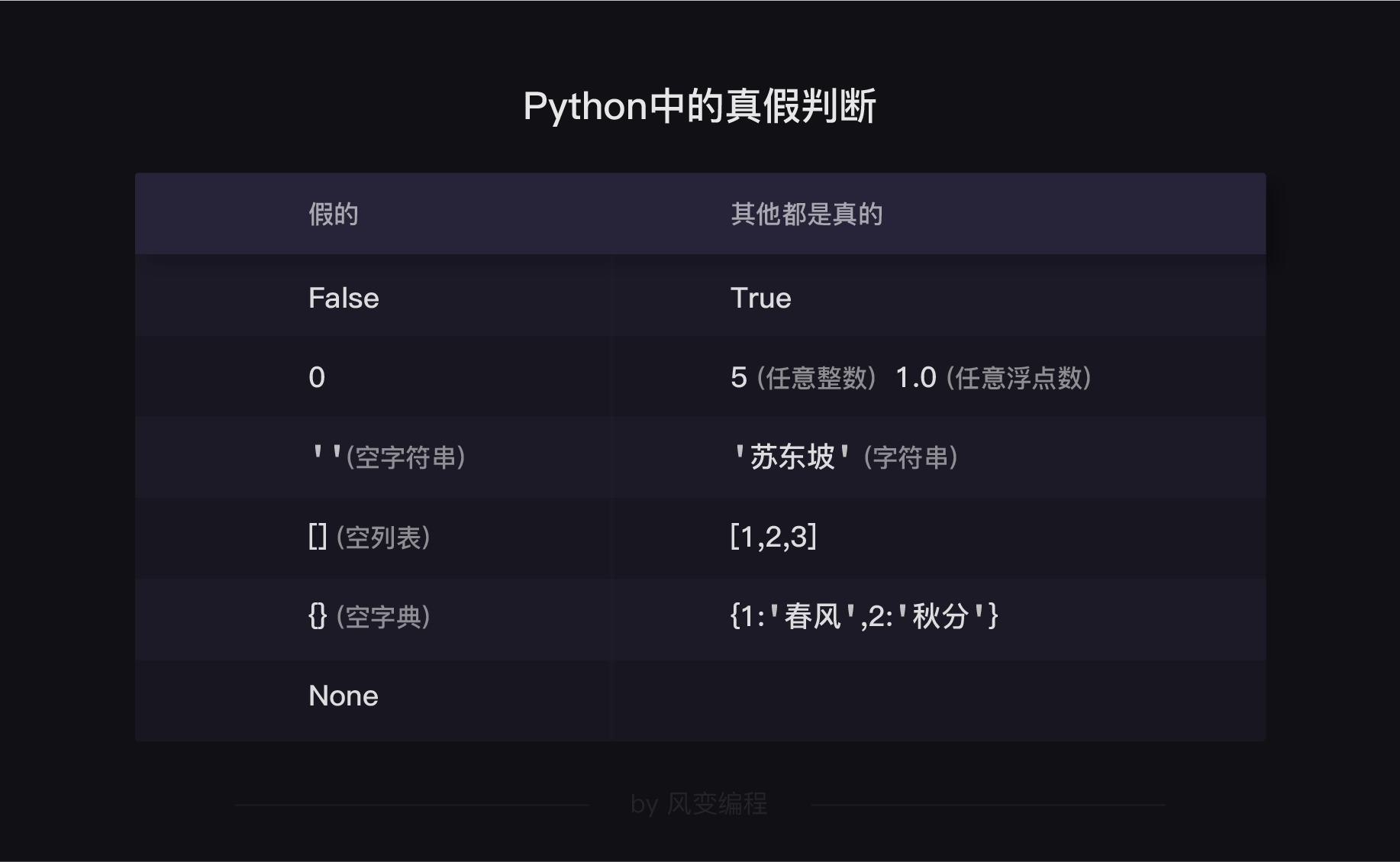``````print('以下数据判断结果都是【假】：')
print(bool(False))
print(bool(0))
print(bool(''))
print(bool(None))

print('以下数据判断结果都是【真】：')
print(bool(True))
print(bool(1))
print(bool('abc'))
``````

bool()函数来查看一个数据会被判断为真还是假。

## 4.布尔值之间的运算

and：与运算，有一个位家，就是假
or：或运算，有一个位真，就是真
not：非运算，真变假，假变真
in：判断一个元素是否在一堆数据之中
not in：判断一个元素是否在一堆数据之中

``````list = [1,2,3,4,5]
a = 1
# 做一次布尔运算，判断“a是否在列表list之中”
print(bool(a in list))
print(bool(a not in list))
``````

【in】和【not in】就可以用来判断字典中是否存在某个【键】：

``````dict = {'法国':'巴黎','日本':'东京','中国':'北京'}
a = '法国'

print(bool(a in dict))
>>>True
``````

## 5.四种新的语句

5.1break语句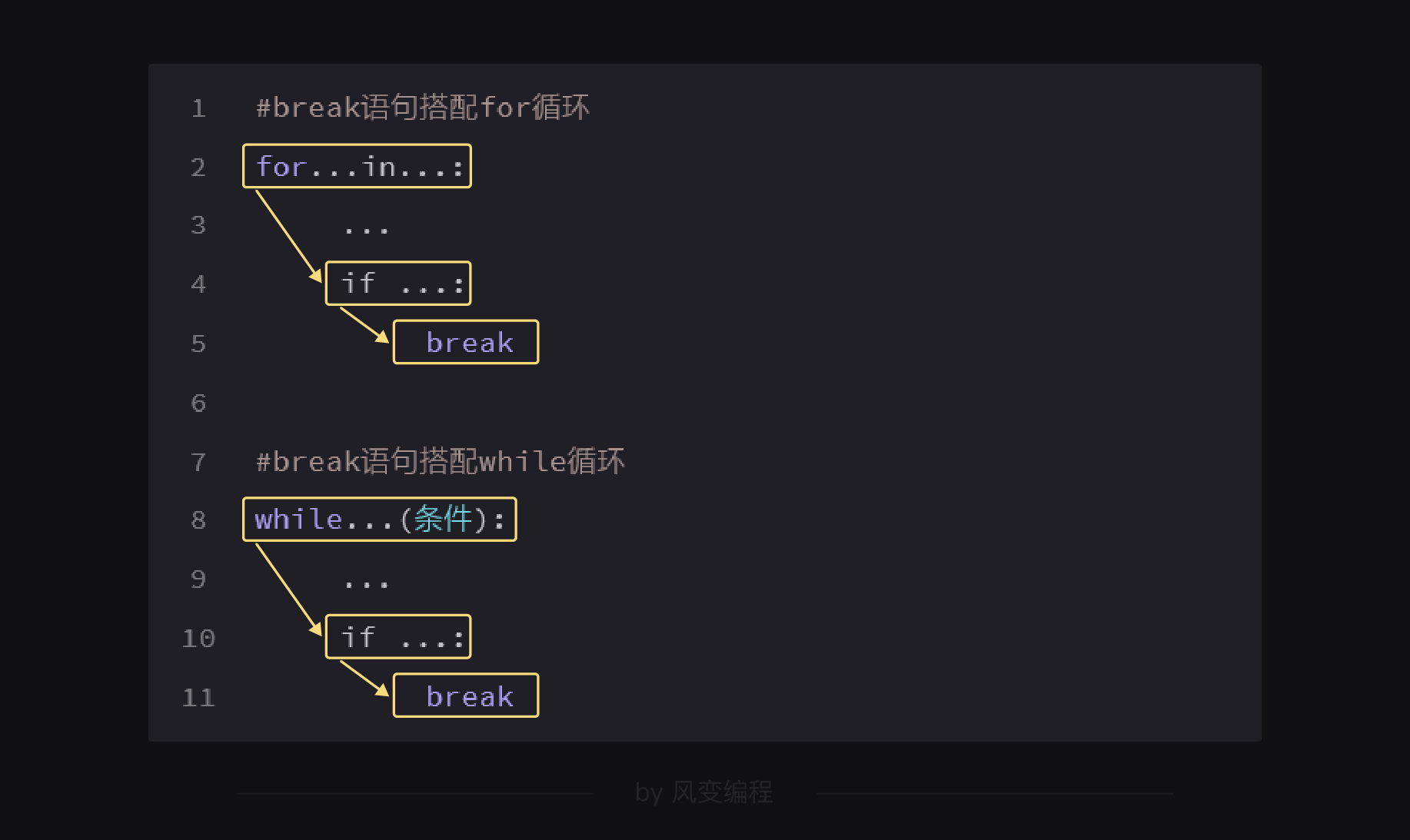if…break的意思是如果满足了某一个条件，就提前结束循环。记住，这个只能在循环内部使用。
5.2 continue语句
continue的意思是“继续”。这个子句也是在循环内部使用的。当某个条件被满足的时候，触发continue语句，将跳过之后的代码，直接回到循环的开始。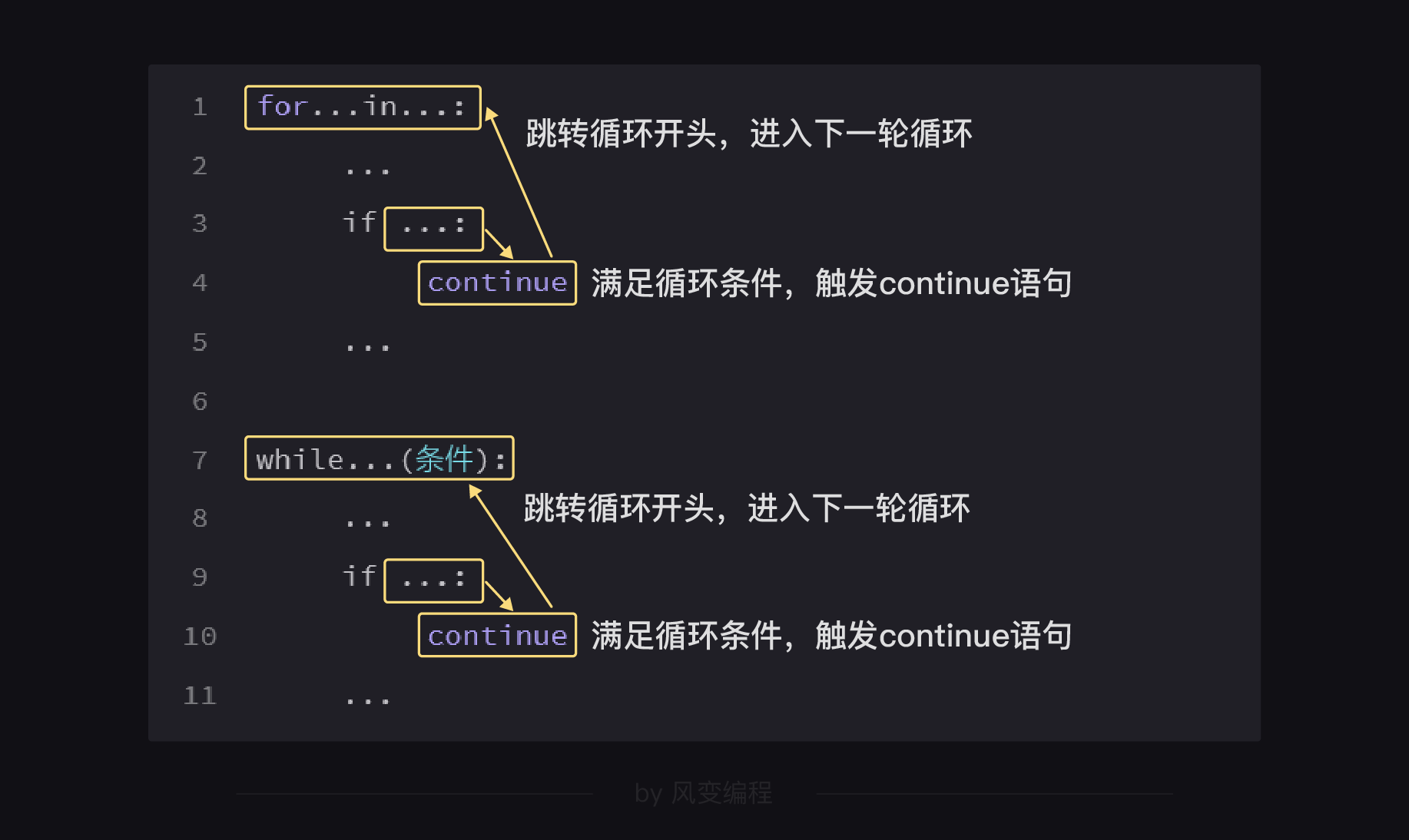``````while True:
q1=input('第一问：你一生之中，在什么地方遇见了喜欢的人？\n')
if q1!='黑暗的冰窖':
continue
print('你答对了第一个问题！请继续回答\n')
q2=input('第二问：你生平最爱之人，叫什么名字？\n')
if q2!='梦姑':
continue
print('你答对了第一个问题！请继续回答\n')
q3=input('第三问：你最爱的这个人长什么样子？\n')
if q3!='不知道':
continue
print('你就是虚竹！')
break
>>>第一问：你一生之中，在什么地方遇见了喜欢的人？

``````

## 5.3pass语句

``````a = int(input('请输入一个整数:'))
if a >= 100:
pass
else:
print('你输入了一个小于100的数字')
``````

## 5.4 else语句

``````i=0
while i!=5:
i=i+1
a = int(input('请输入0来结束循环，你有5次机会:'))
if a == 0:
print('你触发了break语句，循环结束，导致else语句不会生效')
break
else:
print('5次循环你都错过了，else语句生效了。')
``````

``````number1=str(input('输入你心里想的数:'))
for i in range(3):
number2=str(input('输入你猜的数:'))
if number1>number2:
print('你猜的数小了')
elif number1<number2:
print('你猜的数大了')
else:
print('你猜对了，太厉害了')
break
else:
print('你都猜错了')

print('游戏结束')
``````

``````number=0
record=[]
while True:
number+=1
A=input('A你认罪吗，回答认罪或不认罪：')
B=input('B你认罪吗，回答认罪或不认罪：')
record.append([A,B])
if (A=='认罪')and(B=='认罪'):
print('A和B都判10年')
elif (A=='不认罪')and(B=='认罪'):
print('A判20年，B判1年')
elif(A=='认罪')and(B=='不认罪'):
print('A判1年，B判20年')
else:
print('A和B都判3年')
break

print('审判结束')
print('第'+str(number)+'对实验者选了最优解。')
for i in range(number):
print('第'+str(i+1)+'对实验者的选择是：'+str(record[i]))
``````

``````movies = {
'妖猫传':['黄轩','染谷将太'],
'无问西东':['章子怡','王力宏','祖峰'],
'超时空同居':['雷佳音','佟丽娅'],
}

actor=input('输入你要查询的演员：')
for key,value in movies.items():#遍历字典键值对
actors_list=value #将值存起来
for i in actors_list: #遍历值
if i==actor:
print(actor+'出演了电影'+key)
``````

``````movies = {
'妖猫传':['黄轩','染谷将太'],
'无问西东':['章子怡','王力宏','祖峰'],
'超时空同居':['雷佳音','佟丽娅'],
}

actor = input('你想查询哪个演员？')
for i in movies:  # 用 for 遍历字典的键
actors = movies[i]  # 遍历每个键的值
if actor in actors: #判断输入的字符是否在值中
print(actor + '出演了电影' + i)
``````

## 6. for循环遍历字典的四种方法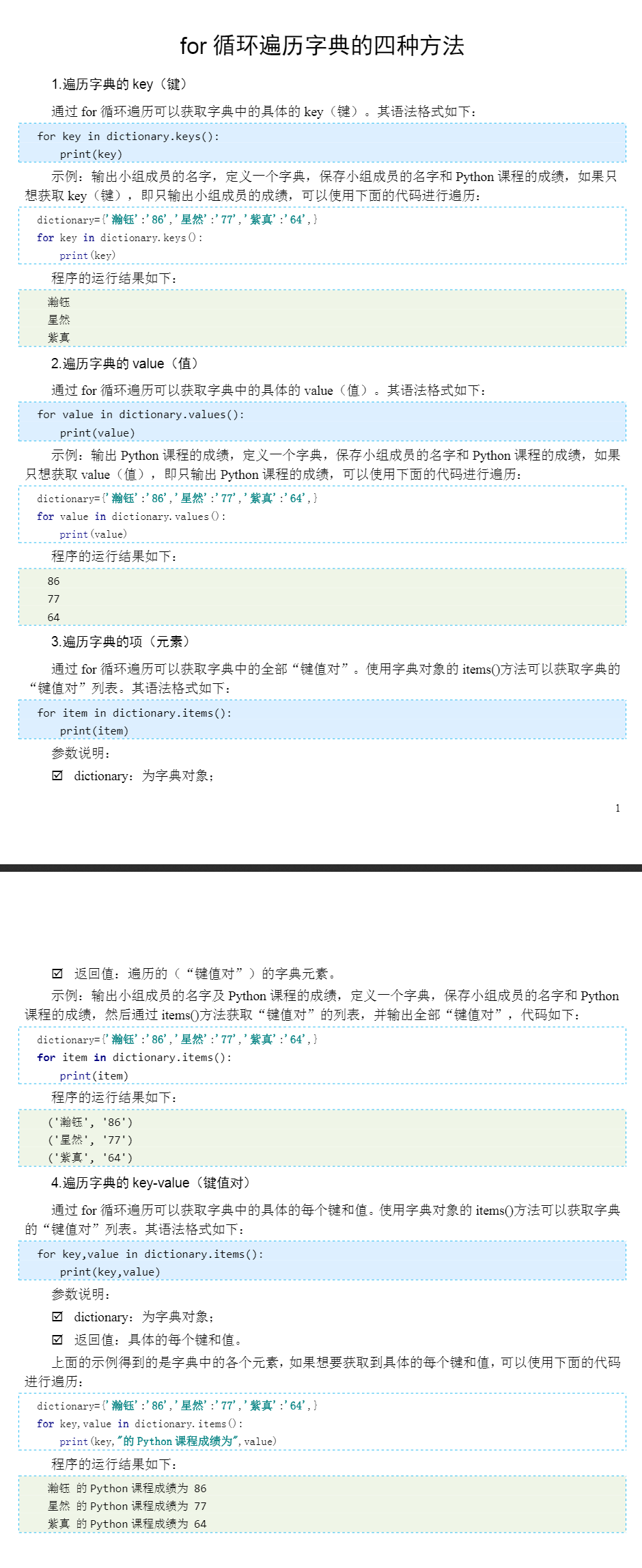15 0

pdf(new) 更多>